Type
Quiz
Book Title
Fundamentals of Corporate Finance Standard Edition 9th Edition
ISBN 13
978-0073382395

### 978-0073382395 Chapter 4 Questions and Problems 21-31

April 3, 2019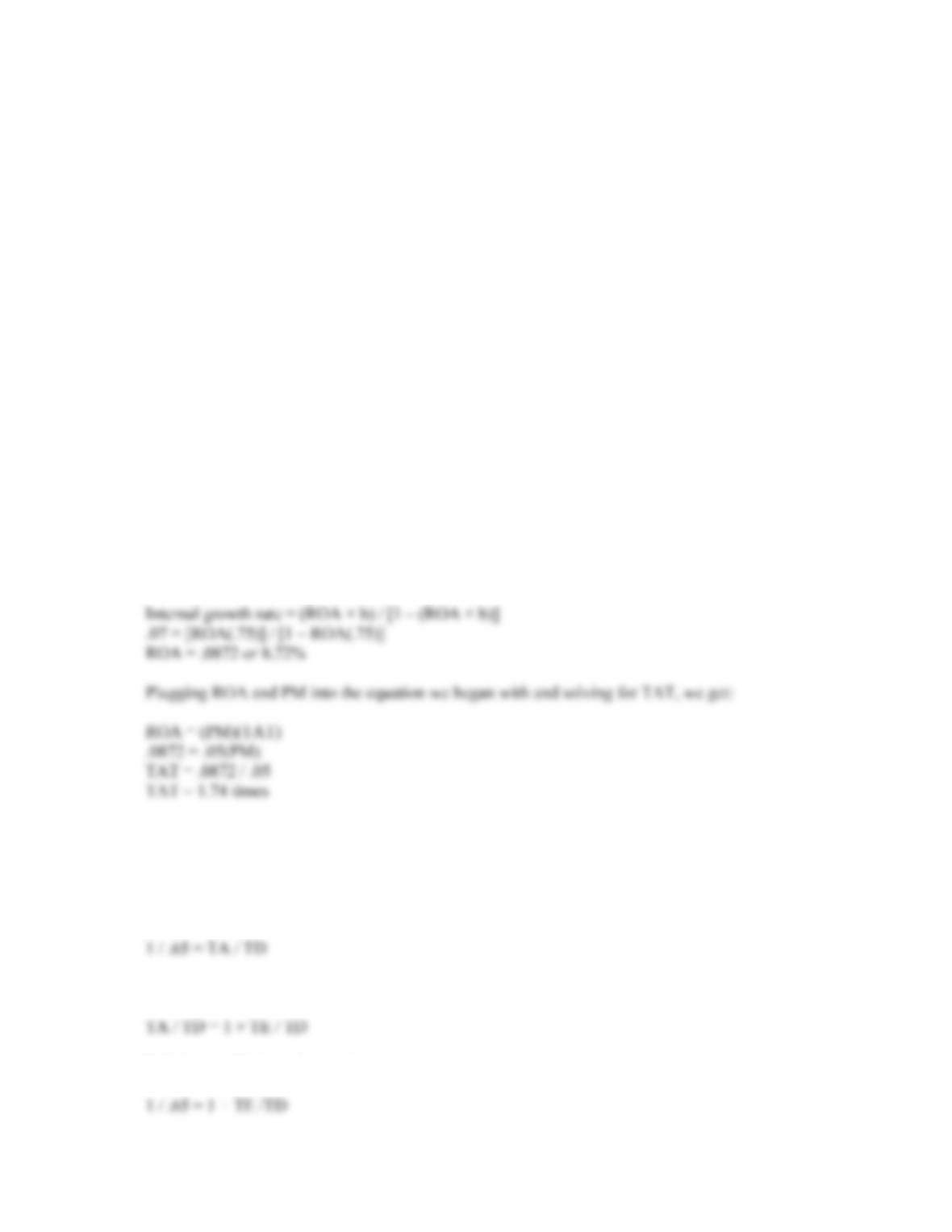CHAPTER 4 B-39
Now we can use the DuPont identity to find the equity multiplier as:
ROE = PM(TAT)(EM)
.1473 = (.062)(1 / .60)EM
EM = (.1473)(.60) / .062
EM = 1.43
So, the D/E ratio is:
D/E = EM – 1
D/E = 1.43 – 1
D/E = 0.43
20. We are given the profit margin. Remember that:
ROA = PM(TAT)
We can calculate the ROA from the internal growth rate formula, and then use the ROA in this
equation to find the total asset turnover. The retention ratio is:
b = 1 – .25
b = .75
Using the internal growth rate equation to find the ROA, we get:
21. We should begin by calculating the D/E ratio. We calculate the D/E ratio as follows:
Total debt ratio = .65 = TD / TA
Inverting both sides we get:
Next, we need to recognize that
Substituting this into the previous equation, we get: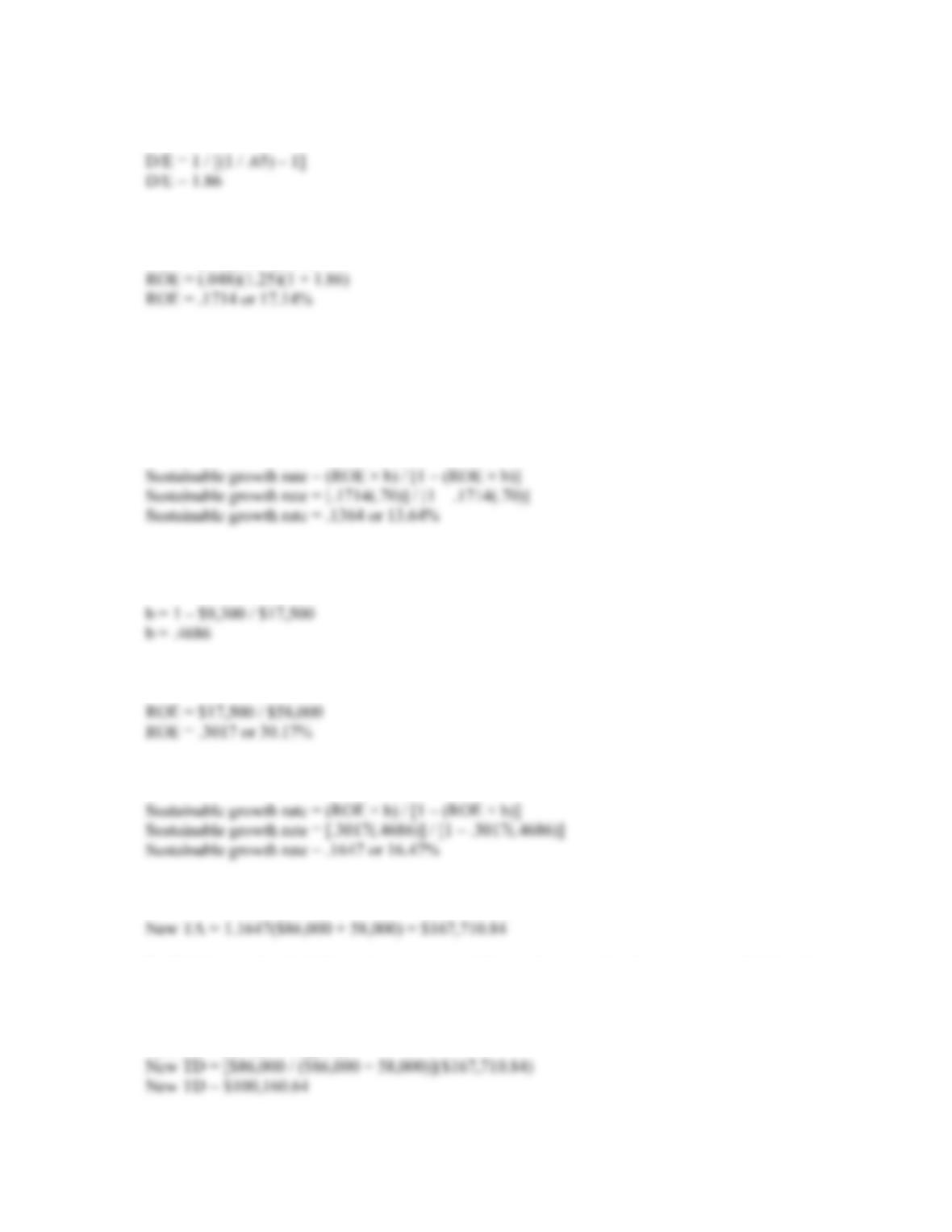B-40 SOLUTIONS
Subtract 1 (one) from both sides and inverting again, we get:
With the D/E ratio, we can calculate the EM and solve for ROE using the DuPont identity:
ROE = (PM)(TAT)(EM)
Now we can calculate the retention ratio as:
b = 1 – .30
b = .70
Finally, putting all the numbers we have calculated into the sustainable growth rate equation, we get:
22. To calculate the sustainable growth rate, we first must calculate the retention ratio and ROE. The
retention ratio is:
And the ROE is:
So, the sustainable growth rate is:
If the company grows at the sustainable growth rate, the new level of total assets is:
To find the new level of debt in the company’s balance sheet, we take the percentage of debt in the
capital structure times the new level of total assets. The additional borrowing will be the new level of
debt minus the current level of debt. So:
New TD = [D / (D + E)](TA)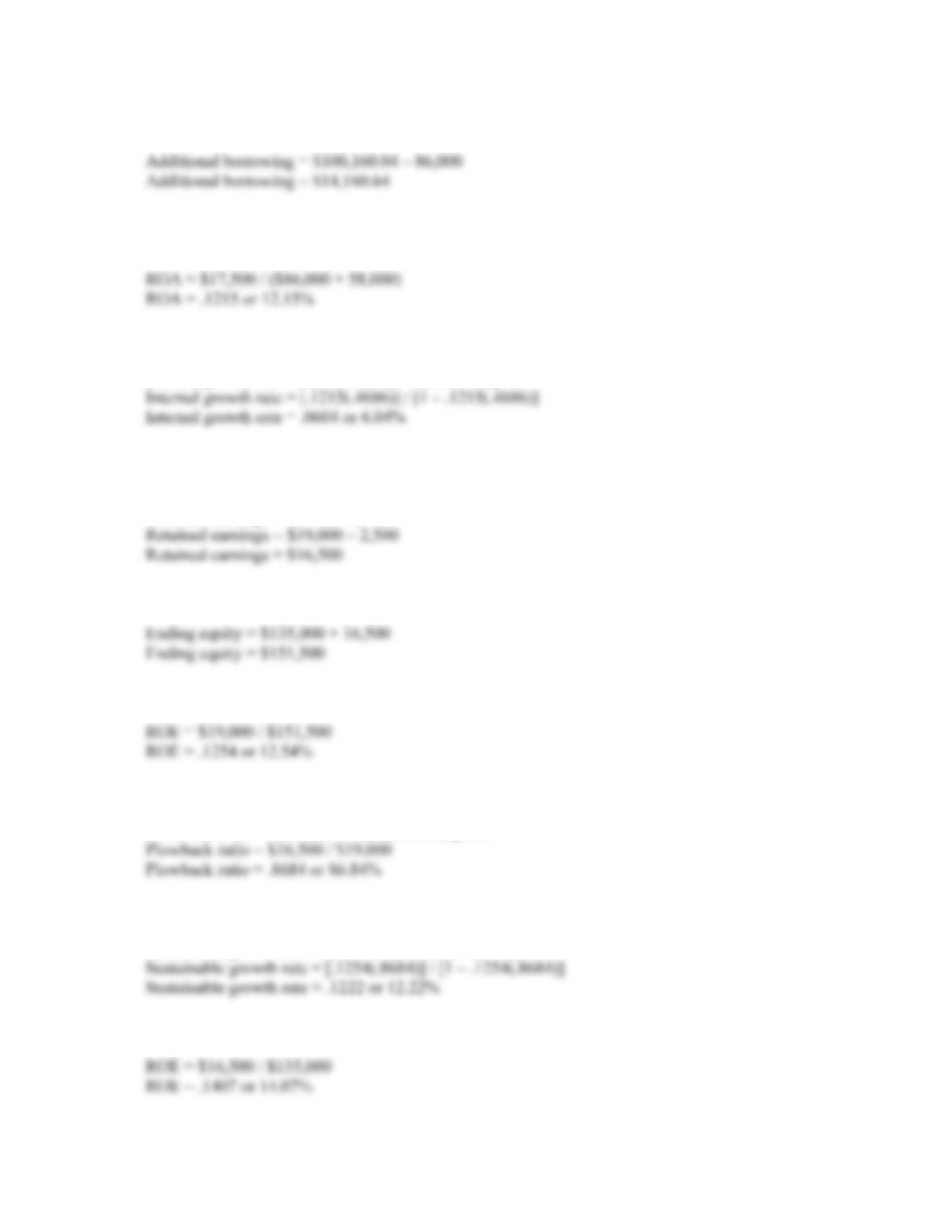CHAPTER 4 B-41
And the additional borrowing will be:
The growth rate that can be supported with no outside financing is the internal growth rate. To
calculate the internal growth rate, we first need the ROA, which is:
This means the internal growth rate is:
Internal growth rate = (ROA × b) / [1 – (ROA × b)]
23. Since the company issued no new equity, shareholders’ equity increased by retained earnings.
Retained earnings for the year were:
Retained earnings = NI – Dividends
So, the equity at the end of the year was:
The ROE based on the end of period equity is:
The plowback ratio is:
Plowback ratio = Addition to retained earnings/NI
Using the equation presented in the text for the sustainable growth rate, we get:
Sustainable growth rate = (ROE × b) / [1 – (ROE × b)]
The ROE based on the beginning of period equity is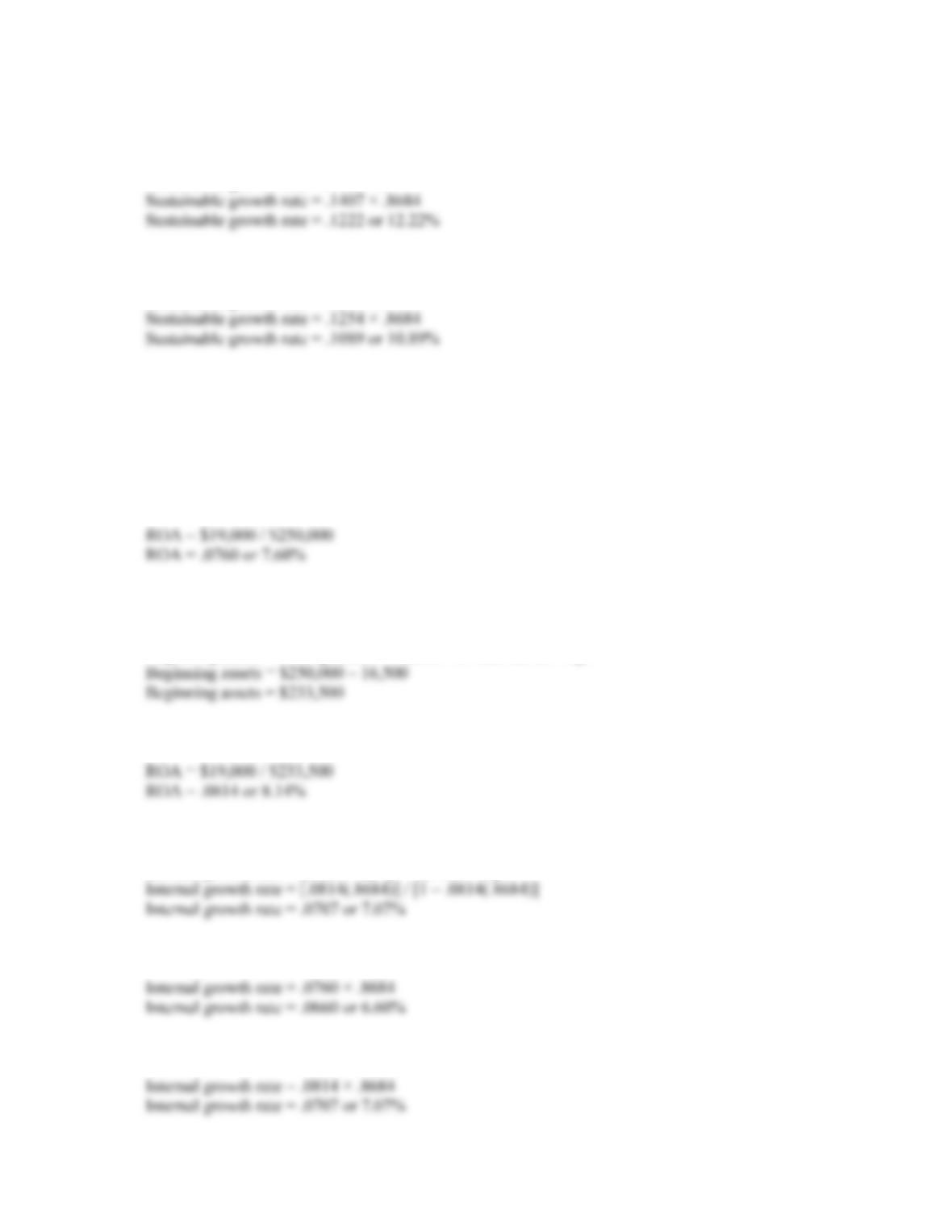B-42 SOLUTIONS
Using the shortened equation for the sustainable growth rate and the beginning of period ROE, we
get:
Sustainable growth rate = ROE × b
Using the shortened equation for the sustainable growth rate and the end of period ROE, we get:
Sustainable growth rate = ROE × b
Using the end of period ROE in the shortened sustainable growth rate results in a growth rate that is
too low. This will always occur whenever the equity increases. If equity increases, the ROE based on
end of period equity is lower than the ROE based on the beginning of period equity. The ROE (and
sustainable growth rate) in the abbreviated equation is based on equity that did not exist when the net
income was earned.
24. The ROA using end of period assets is:
The beginning of period assets had to have been the ending assets minus the addition to retained
earnings, so:
Beginning assets = Ending assets – Addition to retained earnings
And the ROA using beginning of period assets is:
Using the internal growth rate equation presented in the text, we get:
Internal growth rate = (ROA × b) / [1 – (ROA × b)]
Using the formula ROA × b, and end of period assets:
Using the formula ROA × b, and beginning of period assets: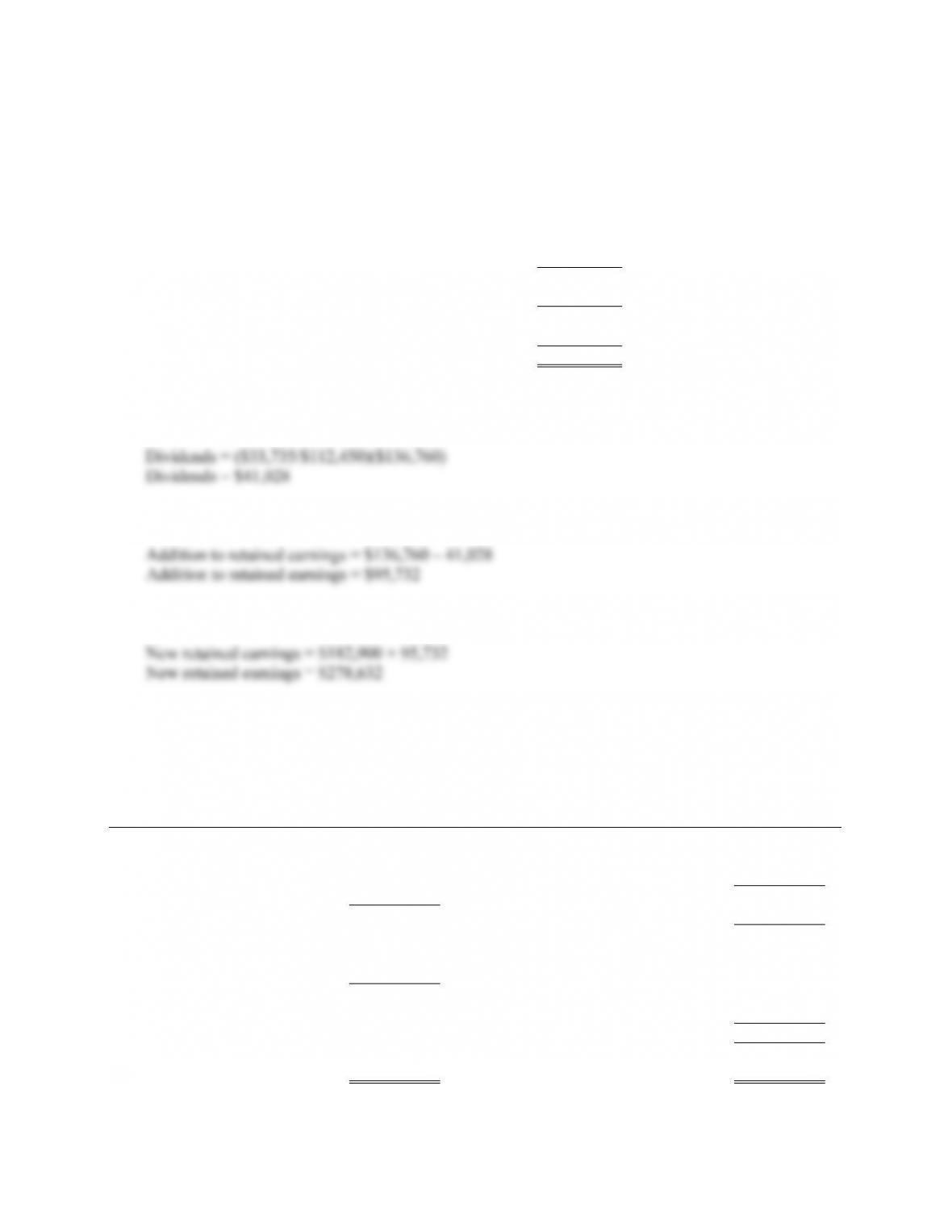CHAPTER 4 B-43
25. Assuming costs vary with sales and a 20 percent increase in sales, the pro forma income statement
will look like this:
MOOSE TOURS INC.
Pro Forma Income Statement
Sales \$ 1,114,800
Costs 867,600
Other expenses 22,800
EBIT \$ 224,400
Interest 14,000
Taxable income \$ 210,400
Taxes(35%) 73,640
Net income \$ 136,760
The payout ratio is constant, so the dividends paid this year is the payout ratio from last year times
net income, or:
And the addition to retained earnings will be:
The new retained earnings on the pro forma balance sheet will be:
The pro forma balance sheet will look like this:
MOOSE TOURS INC.
Pro Forma Balance Sheet
Assets Liabilities and Owners’ Equity
Current assets Current liabilities
Cash \$ 30,360 Accounts payable \$ 81,600
Accounts receivable 48,840 Notes payable 17,000
Inventory 104,280 Total \$ 98,600
Total \$ 183,480 Long-term debt 158,000
Fixed assets
Net plant and Owners’ equity
equipment 495,600 Common stock and
paid-in surplus \$ 140,000
Retained earnings 278,632
Total \$ 418,632
Total liabilities and owners’
Total assets \$ 679,080 equity \$ 675,232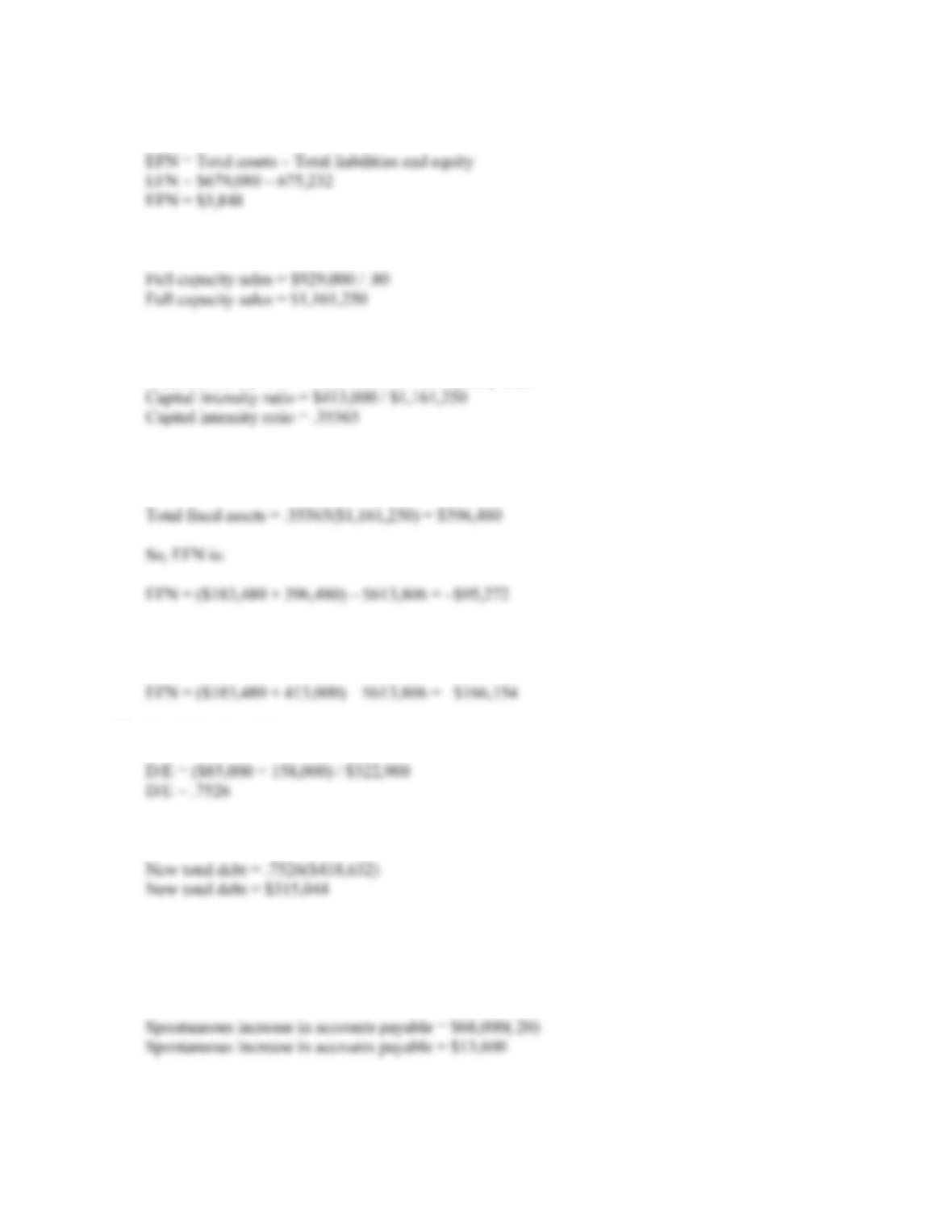B-44 SOLUTIONS
So the EFN is:
26. First, we need to calculate full capacity sales, which is:
The capital intensity ratio at full capacity sales is:
Capital intensity ratio = Fixed assets / Full capacity sales
The fixed assets required at full capacity sales is the capital intensity ratio times the projected sales
level:
Note that this solution assumes that fixed assets are decreased (sold) so the company has a 100
percent fixed asset utilization. If we assume fixed assets are not sold, the answer becomes:
27. The D/E ratio of the company is:
So the new total debt amount will be:
This is the new total debt for the company. Given that our calculation for EFN is the amount that
must be raised externally and does not increase spontaneously with sales, we need to subtract the
spontaneous increase in accounts payable. The new level of accounts payable will be, which is the
current accounts payable times the sales growth, or: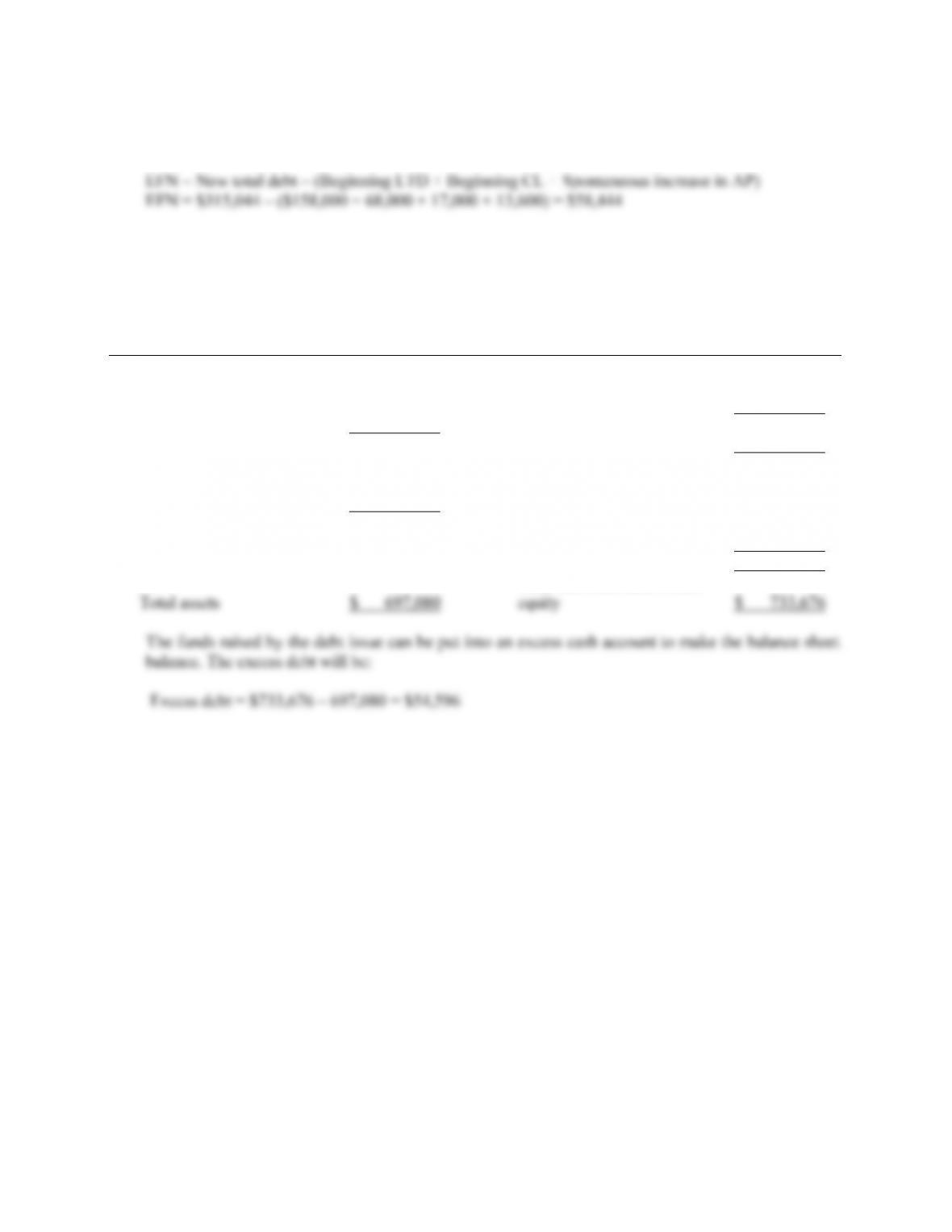CHAPTER 4 B-45
This means that \$13,600 of the new total debt is not raised externally. So, the debt raised externally,
which will be the EFN is:
The pro forma balance sheet with the new long-term debt will be:
MOOSE TOURS INC.
Pro Forma Balance Sheet
Assets Liabilities and Owners’ Equity
Current assets Current liabilities
Cash \$ 30,360 Accounts payable \$ 81,600
Accounts receivable 44,400 Notes payable 17,000
Inventory 104,280 Total \$ 98,600
Total \$ 183,480 Long-term debt 216,444
Fixed assets
Net plant and Owners’ equity
equipment 495,600 Common stock and
paid-in surplus \$ 140,000
Retained earnings 278,632
Total \$ 418,632
Total liabilities and owners’
To make the balance sheet balance, the company will have to increase its assets. We will put this
amount in an account called excess cash, which will give us the following balance sheet: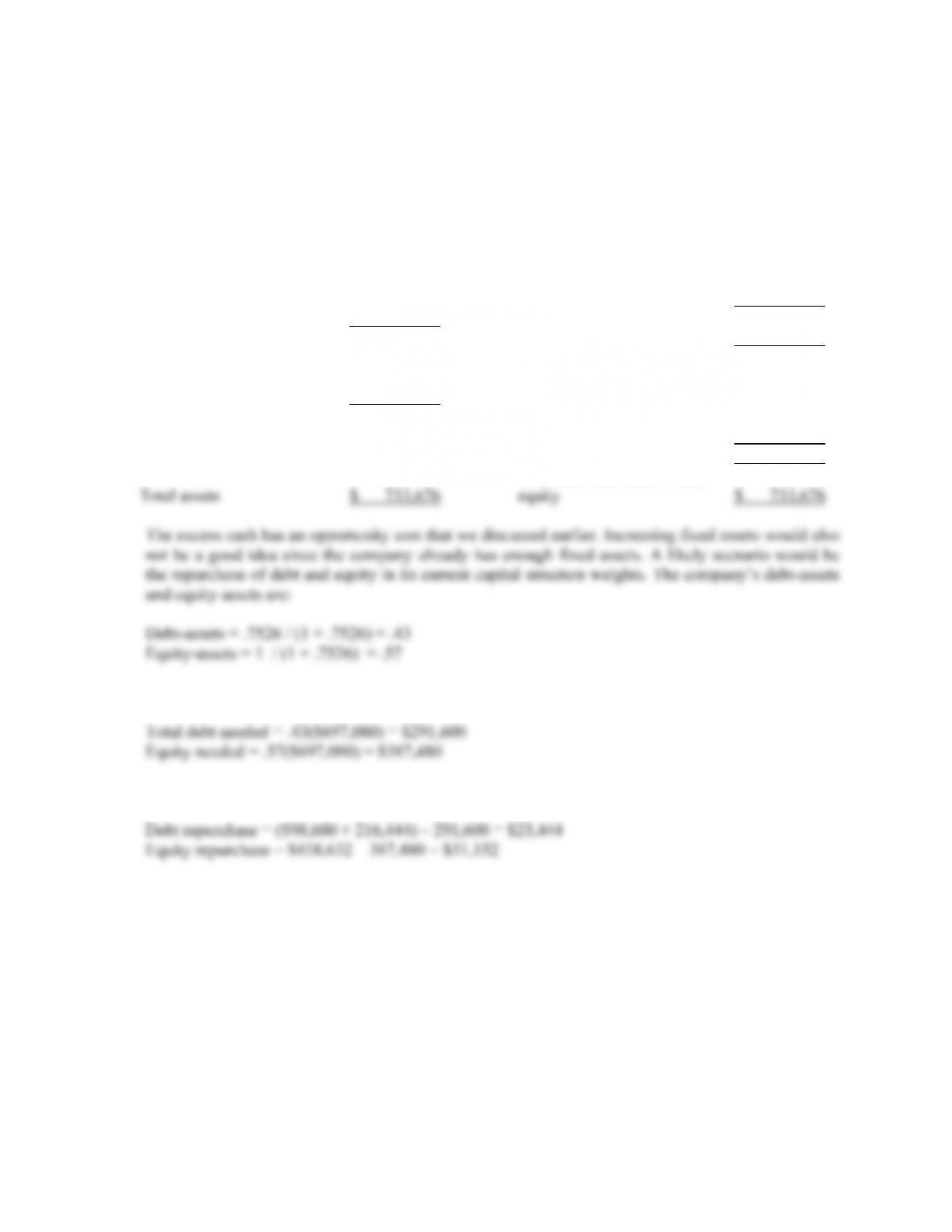B-46 SOLUTIONS
MOOSE TOURS INC.
Pro Forma Balance Sheet
Assets Liabilities and Owners’ Equity
Current assets Current liabilities
Cash \$ 30,360 Accounts payable \$ 81,600
Excess cash 54,596
Accounts receivable 44,400 Notes payable 17,000
Inventory 104,280 Total \$ 98,600
Total \$ 238,076 Long-term debt 216,444
Fixed assets
Net plant and Owners’ equity
equipment 495,600 Common stock and
paid-in surplus \$ 140,000
Retained earnings 278,632
Total \$ 418,632
Total liabilities and owners’
So, the amount of debt and equity needed will be:
So, the repurchases of debt and equity will be:
Assuming all of the debt repurchase is from long-term debt, and the equity repurchase is entirely
from the retained earnings, the final pro forma balance sheet will be: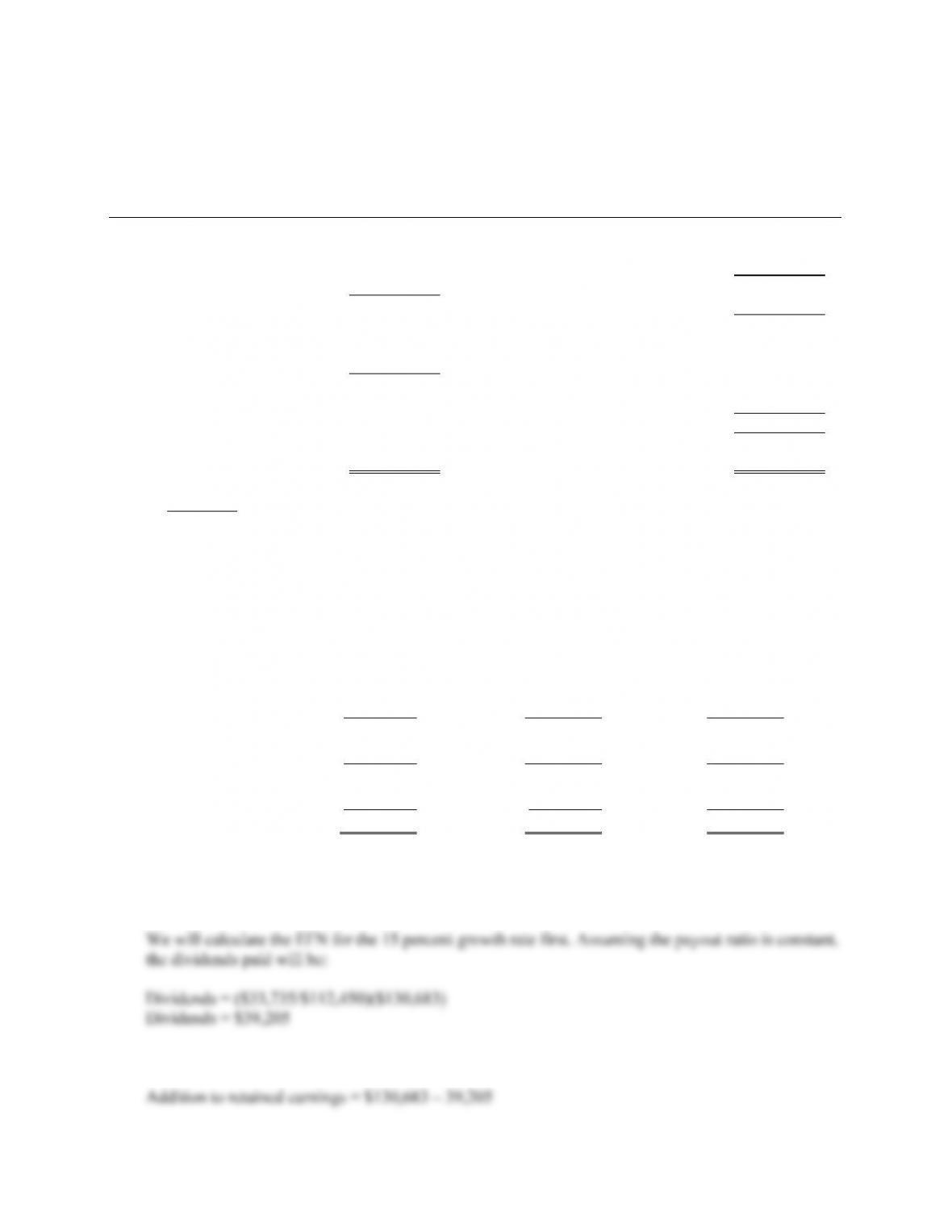CHAPTER 4 B-47
MOOSE TOURS INC.
Pro Forma Balance Sheet
Assets Liabilities and Owners’ Equity
Current assets Current liabilities
Cash \$ 30,360 Accounts payable \$ 81,600
Accounts receivable 44,400 Notes payable 17,000
Inventory 104,280 Total \$ 98,600
Total \$ 183,480 Long-term debt 193,000
Fixed assets
Net plant and Owners’ equity
equipment 495,600 Common stock and
paid-in surplus \$ 140,000
Retained earnings 247,480
Total \$ 387,480
Total liabilities and owners’
Total assets \$ 697,080 equity \$ 697,080
Challenge
28. The pro forma income statements for all three growth rates will be:
MOOSE TOURS INC.
Pro Forma Income Statement
15 % Sales
Growth 20% Sales
Growth 25% Sales
Growth
Sales \$1,068,350 \$1,114,800 \$1,161,250
Costs 831,450 867,600 903,750
Other expenses 21,850 22,800 23,750
EBIT \$215,050 \$224,400 \$233,750
Interest 14,000 14,000 14,000
Taxable income \$201,050 \$210,400 \$219,750
Taxes (35%) 70,368 73,640 76,913
N
et income \$130,683 \$136,760 \$142,838
Dividends \$39,205 \$41,028 \$42,851
Add to RE 91,478 95,732 99,986
And the addition to retained earnings will be: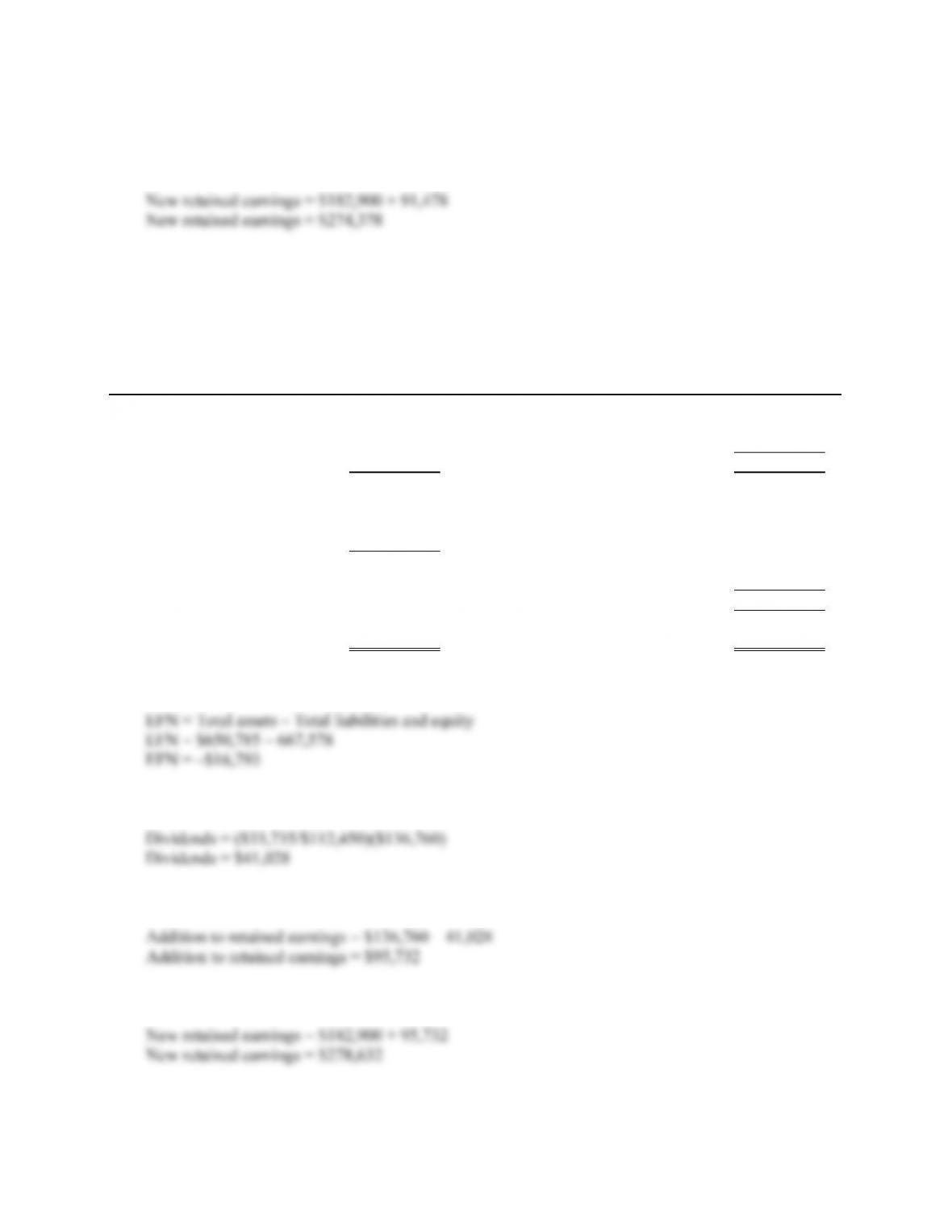B-48 SOLUTIONS
Addition to retained earnings = \$91,478
The new retained earnings on the pro forma balance sheet will be:
The pro forma balance sheet will look like this:
15% Sales Growth:
MOOSE TOURS INC.
Pro Forma Balance Sheet
Assets Liabilities and Owners’ Equity
Current assets Current liabilities
Cash \$ 29,095 Accounts payable \$ 78,200
Accounts receivable 46,805 Notes payable 17,000
Inventory 99,935 Total \$ 95,200
Total \$ 175,835 Long-term debt \$ 158,000
Fixed assets
Net plant and Owners’ equity
equipment 474,950 Common stock and
paid-in surplus \$ 140,000
Retained earnings 274,378
Total \$ 414,378
Total liabilities and owners’
Total assets \$ 650,785 equity \$ 667,578
So the EFN is:
At a 20 percent growth rate, and assuming the payout ratio is constant, the dividends paid will be:
And the addition to retained earnings will be:
The new retained earnings on the pro forma balance sheet will be: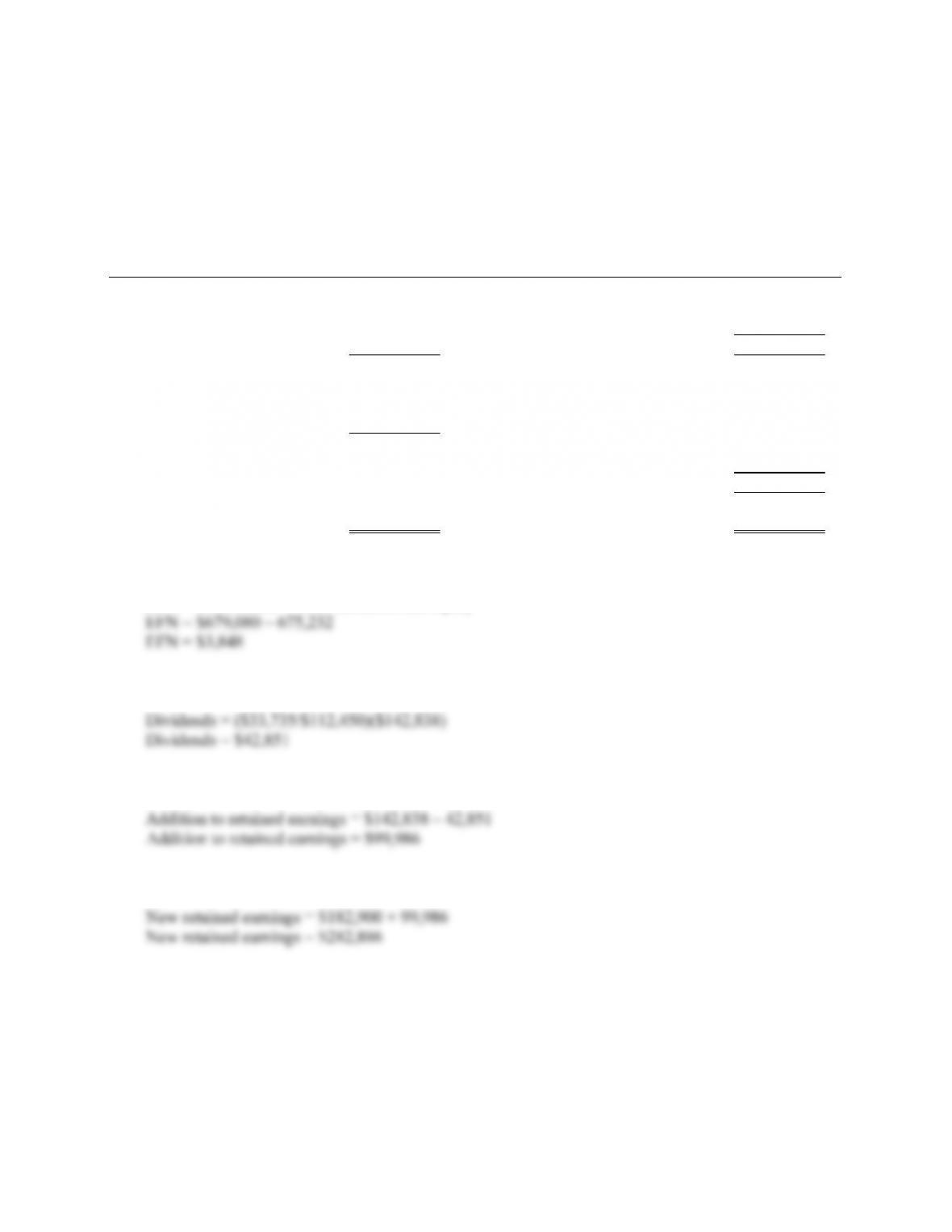CHAPTER 4 B-49
The pro forma balance sheet will look like this:
20% Sales Growth:
MOOSE TOURS INC.
Pro Forma Balance Sheet
Assets Liabilities and Owners’ Equity
Current assets Current liabilities
Cash \$ 30,360 Accounts payable \$ 81,600
Accounts receivable 48,840 Notes payable 17,000
Inventory 104,280 Total \$ 98,600
Total \$ 183,480 Long-term debt \$ 158,000
Fixed assets
Net plant and Owners’ equity
equipment 495,600 Common stock and
paid-in surplus \$ 140,000
Retained earnings 278,632
Total \$ 418,632
Total liabilities and owners’
Total assets \$ 679,080 equity \$ 675,232
So the EFN is:
EFN = Total assets – Total liabilities and equity
At a 25 percent growth rate, and assuming the payout ratio is constant, the dividends paid will be:
And the addition to retained earnings will be:
The new retained earnings on the pro forma balance sheet will be:
The pro forma balance sheet will look like this: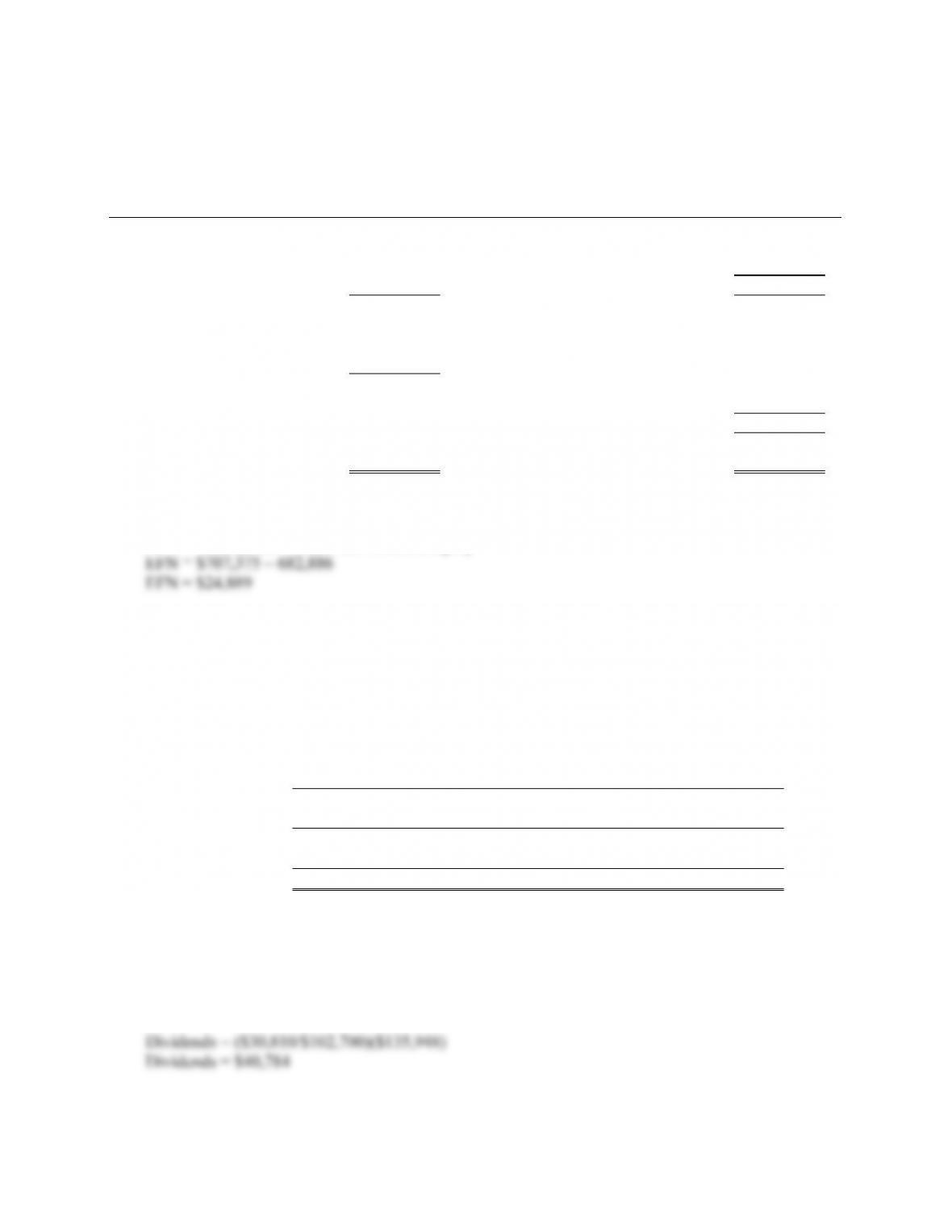B-50 SOLUTIONS
25% Sales Growth:
MOOSE TOURS INC.
Pro Forma Balance Sheet
Assets Liabilities and Owners’ Equity
Current assets Current liabilities
Cash \$ 31,625 Accounts payable \$ 85,000
Accounts receivable 50,875 Notes payable 17,000
Inventory 108,625 Total \$ 102,000
Total \$ 191,125 Long-term debt \$ 158,000
Fixed assets
Net plant and Owners’ equity
equipment 516,250 Common stock and
paid-in surplus \$ 140,000
Retained earnings 282,886
Total \$ 422,886
Total liabilities and owners’
Total assets \$ 707,375 equity \$ 682,886
So the EFN is:
EFN = Total assets – Total liabilities and equity
29. The pro forma income statements for all three growth rates will be:
MOOSE TOURS INC.
Pro Forma Income Statement
20% Sales
Growth 30% Sales
Growth 35% Sales
Growth
Sales \$1,114,800 \$1,207,700 \$1,254,150
Costs 867,600 939,900 976,050
Other expenses 22,800 24,700 25,650
EBIT \$224,400 \$243,100 \$252,450
Interest 14,000 14,000 14,000
Taxable income \$210,400 \$229,100 \$238,450
Taxes (35%) 73,640 80,185 83,458
N
et income \$136,760 \$148,915 \$154,993
Dividends \$41,028 \$44,675 \$46,498
Add to RE 95,732 104,241 108,495
At a 30 percent growth rate, and assuming the payout ratio is constant, the dividends paid will be:
And the addition to retained earnings will be: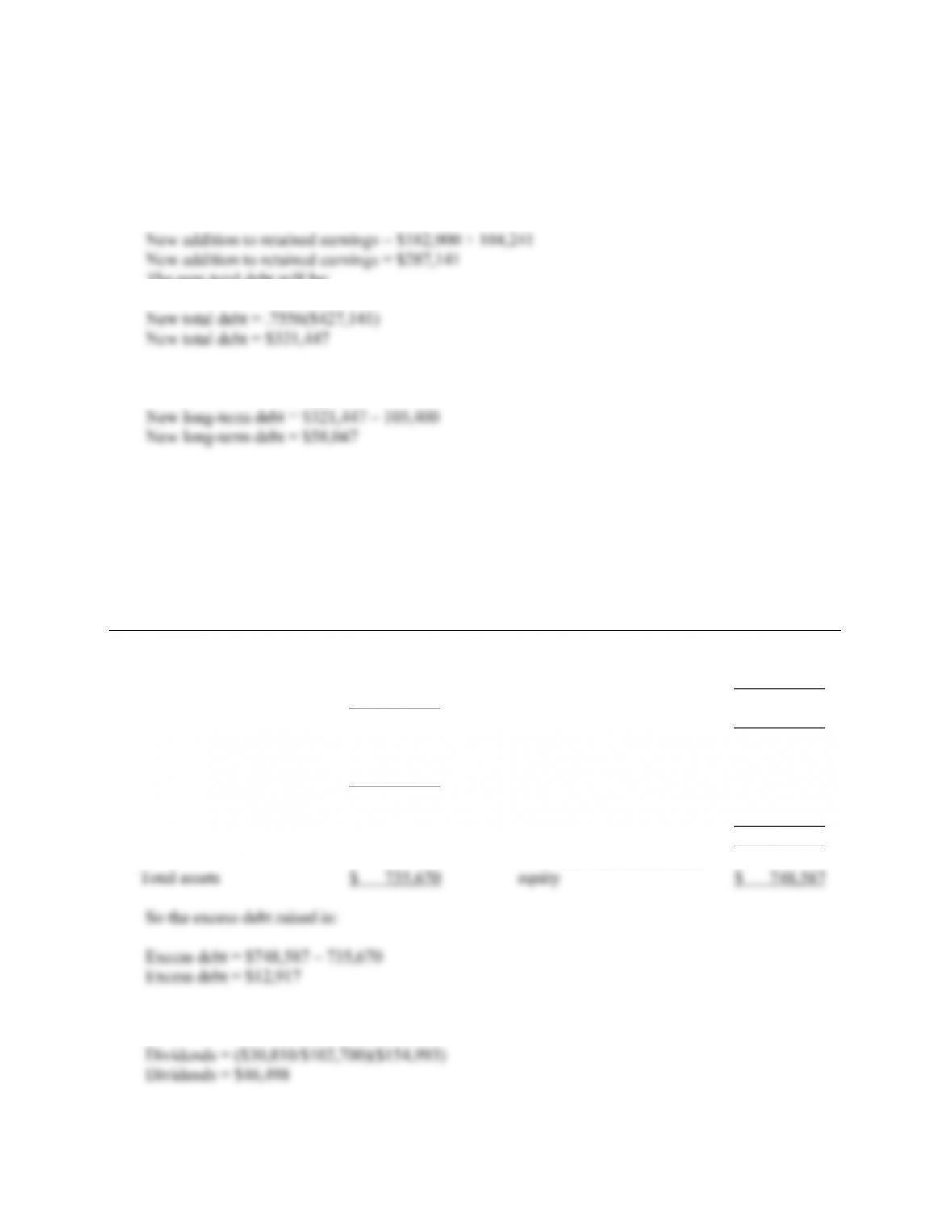CHAPTER 4 B-51
Addition to retained earnings = \$135,948 – 40,784
Addition to retained earnings = \$104,241
The new addition to retained earnings on the pro forma balance sheet will be:
The new total debt will be:
So, the new long-term debt will be the new total debt minus the new short-term debt, or:
The pro forma balance sheet will look like this:
Sales growth rate = 30% and debt/equity ratio = .7526:
MOOSE TOURS INC.
Pro Forma Balance Sheet
Assets Liabilities and Owners’ Equity
Current assets Current liabilities
Cash \$ 32,890 Accounts payable \$ 88,400
Accounts receivable 52,910 Notes payable 17,000
Inventory 112,970 Total \$ 105,400
Total \$ 198,770 Long-term debt 216,047
Fixed assets
Net plant and Owners’ equity
equipment 536,900 Common stock and
paid-in surplus \$ 140,000
Retained earnings 287,141
Total \$ 427,141
Total liabilities and owners’
At a 35 percent growth rate, and assuming the payout ratio is constant, the dividends paid will be: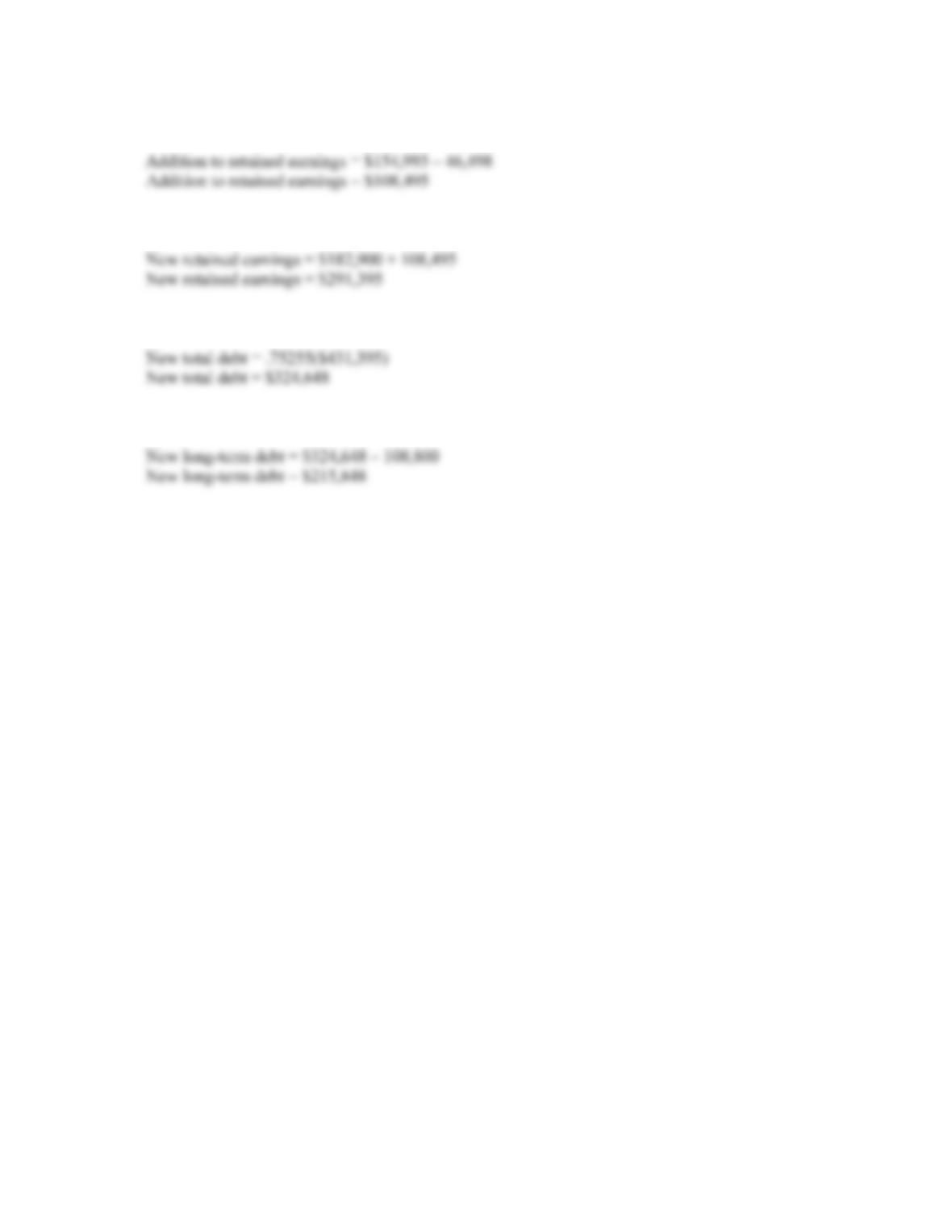B-52 SOLUTIONS
And the addition to retained earnings will be:
The new retained earnings on the pro forma balance sheet will be:
The new total debt will be:
So, the new long-term debt will be the new total debt minus the new short-term debt, or: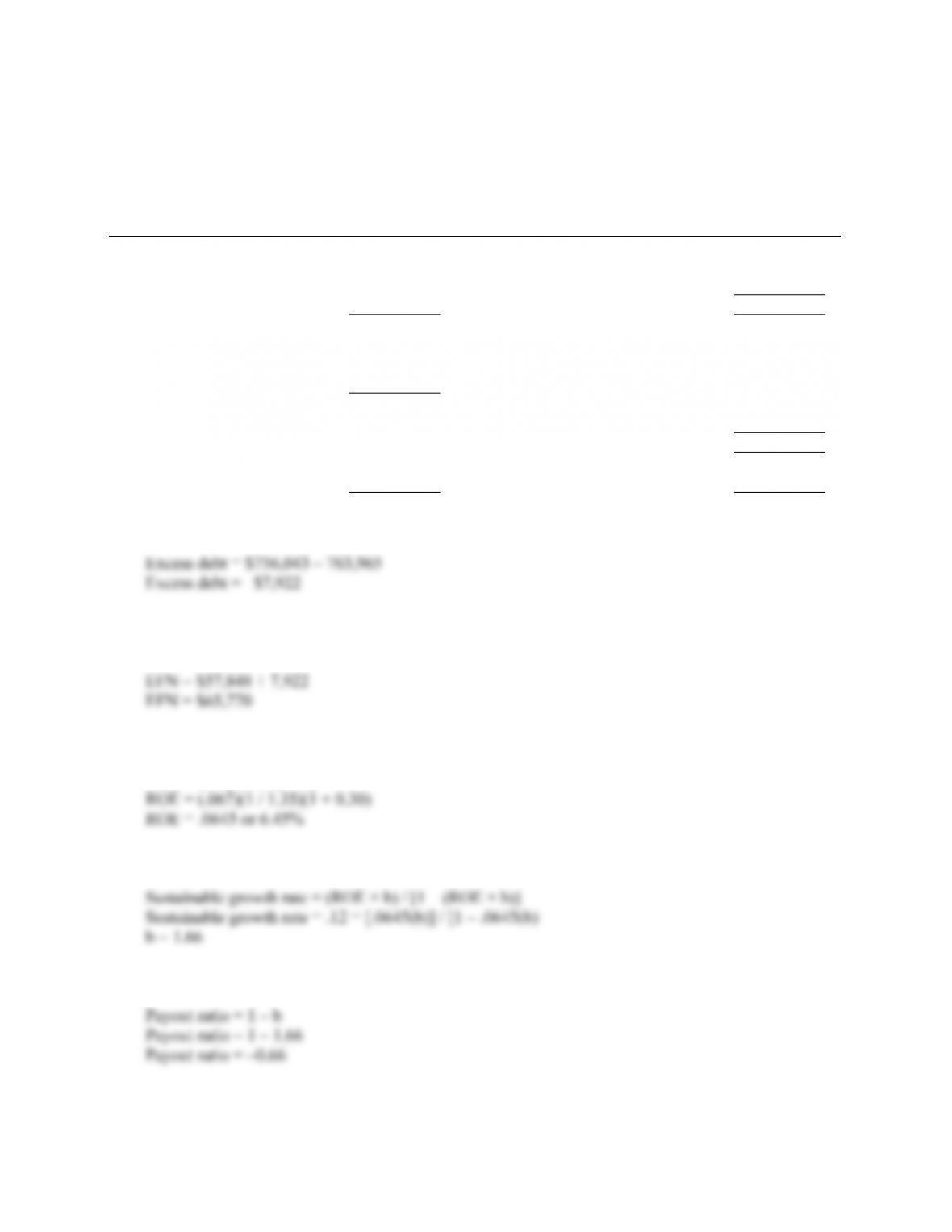CHAPTER 4 B-53
Sales growth rate = 35% and debt/equity ratio = .75255:
MOOSE TOURS INC.
Pro Forma Balance Sheet
Assets Liabilities and Owners’ Equity
Current assets Current liabilities
Cash \$ 34,155 Accounts payable \$ 91,800
Accounts receivable 54,945 Notes payable 17,000
Inventory 117,315 Total \$ 108,800
Total \$ 206,415 Long-term debt \$ 215,848
Fixed assets
Net plant and Owners’ equity
equipment 557,550 Common stock and
paid-in surplus \$ 140,000
Retained earnings 291,395
Total \$ 431,395
Total liabilities and owners’
Total assets \$ 763,965 equity \$ 756,043
So the excess debt raised is:
At a 35 percent growth rate, the firm will need funds in the amount of \$7,922 in addition to the
external debt already raised. So, the EFN will be:
30. We must need the ROE to calculate the sustainable growth rate. The ROE is:
ROE = (PM)(TAT)(EM)
Now we can use the sustainable growth rate equation to find the retention ratio as:
This implies the payout ratio is: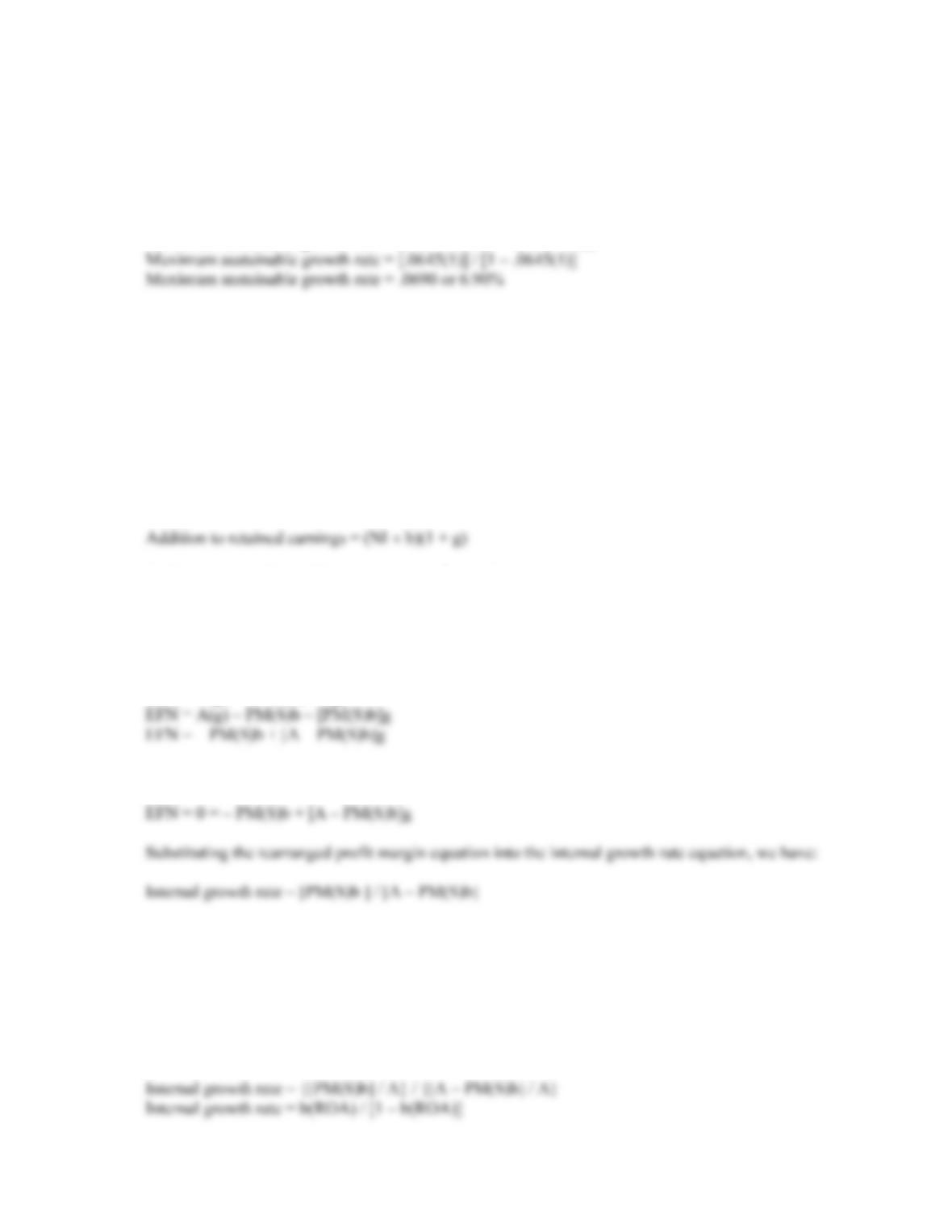B-54 SOLUTIONS
This is a negative dividend payout ratio of 66 percent, which is impossible. The growth rate is not
consistent with the other constraints. The lowest possible payout rate is 0, which corresponds to
retention ratio of 1, or total earnings retention.
The maximum sustainable growth rate for this company is:
Maximum sustainable growth rate = (ROE × b) / [1 – (ROE × b)]
31. We know that EFN is:
EFN = Increase in assets – Addition to retained earnings
The increase in assets is the beginning assets times the growth rate, so:
Increase in assets = A g
The addition to retained earnings next year is the current net income times the retention ratio, times
one plus the growth rate, so:
And rearranging the profit margin to solve for net income, we get:
NI = PM(S)
Substituting the last three equations into the EFN equation we started with and rearranging, we get:
EFN = A(g) – PM(S)b(1 + g)
32. We start with the EFN equation we derived in Problem 31 and set it equal to zero:
Since:
ROA = NI / A
ROA = PM(S) / A
We can substitute this into the internal growth rate equation and divide both the numerator and
denominator by A. This gives: